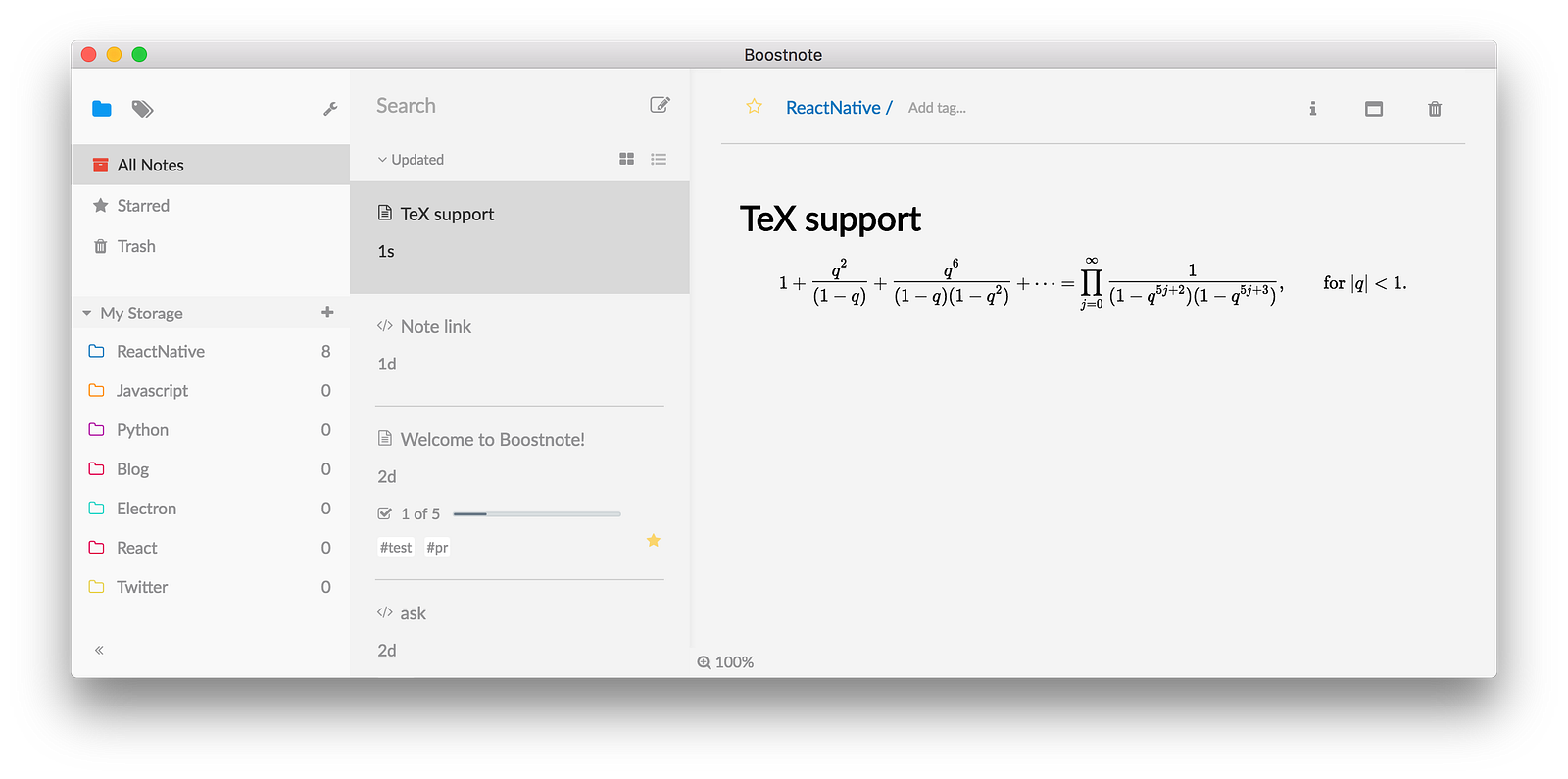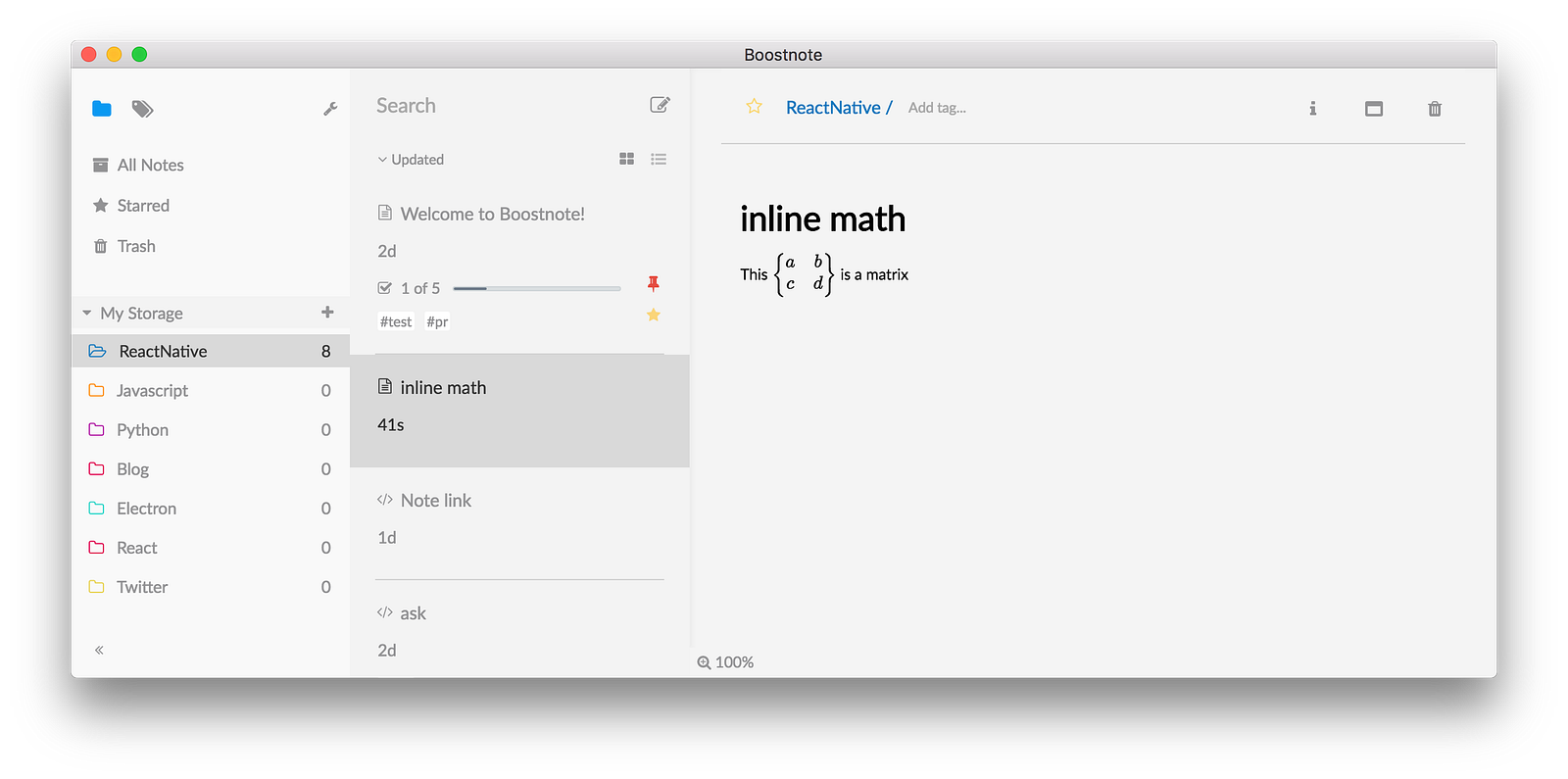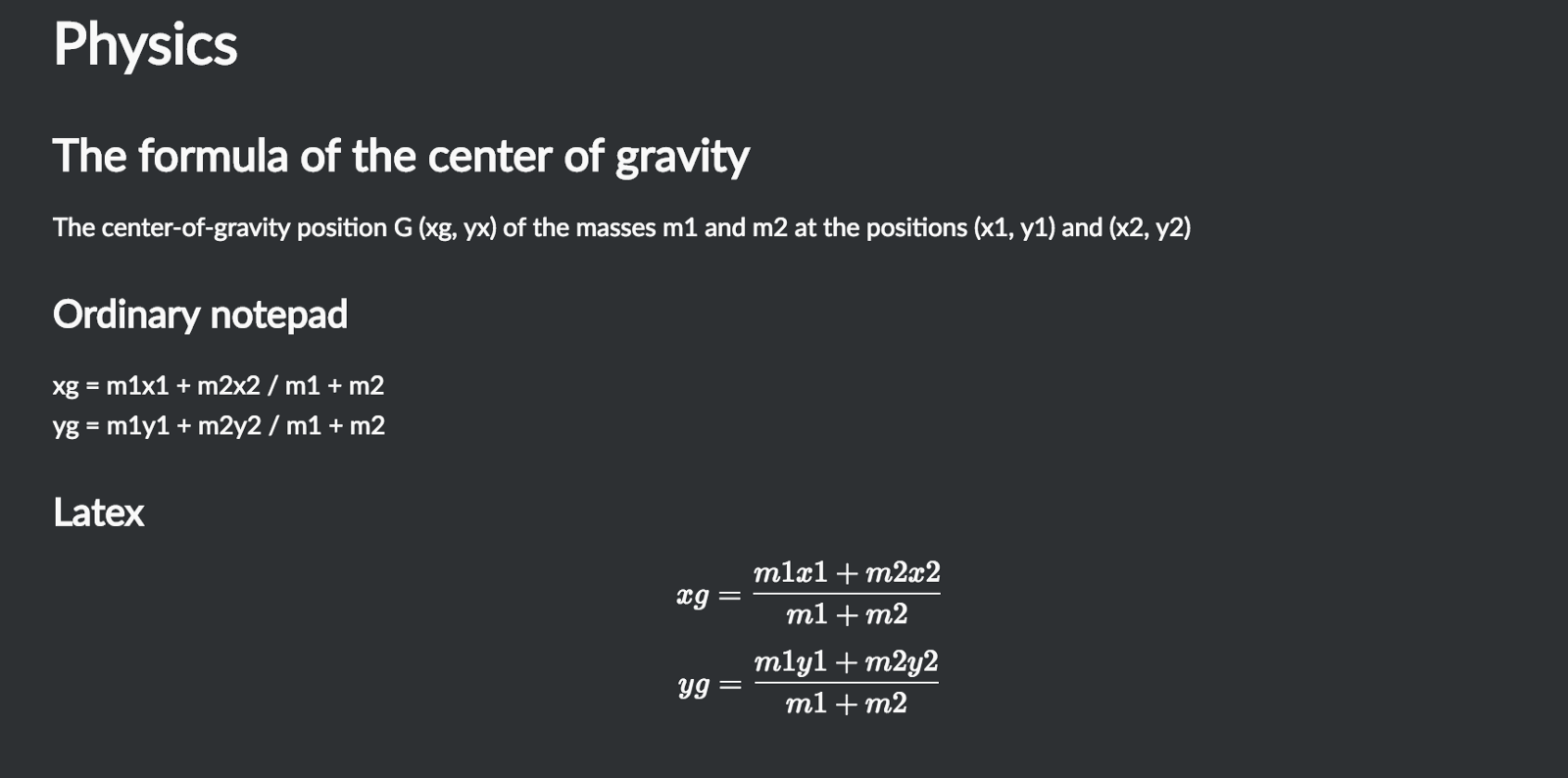# How to write math formulas beautifully using TeX.## What is TeX?

TeX (/ˈtɛx/ or /ˈtɛk/, see below), stylized within the system as TEX, is a typesetting system (or “formatting system”) designed and mostly written by Donald Knuth and released in 1978. Together with the Metafont language for fontdescription and the Computer Modern family of typefaces, TeX was designed with two main goals in mind: to allow anybody to produce high-quality books using minimal effort, and to provide a system that would give exactly the same results on all computers, at any point in time. TeX is free software, which made it accessible to a wide range of users. ref: https://en.wikipedia.org/wiki/TeX

## TeX support

You can write mathematics using TeX such as $$and$$$. $$1 + \frac{q²}{(1-q)}+\frac{q⁶}{(1-q)(1-q²)}+\cdots = \prod_{j=0}^{\infty}\frac{1}{(1-q^{5j+2})(1-q^{5j+3})}, \quad\quad \text{for }\lvert q\rvert<1.$$$## Inline math

This $$\begin{Bmatrix} a & b \\ c & d \end{Bmatrix}$$ is a matrixI drew the formula of physics. It’s overwhelming easier to see compared to taking notes normally.This is useful for study, research and more.

Enjoy TeX with Boostnote✌️

## READ NEXT

Boostlog is an online community for developers
who want to share ideas and grow each other.

# Delete an article

Deleted articles are gone forever. Are you sure?